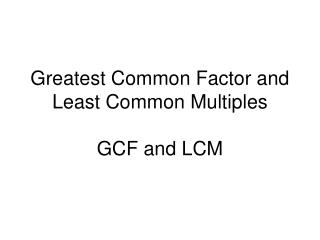DownloadDownload PresentationGreatest Common Factor and Least Common Multiples GCF and LCM

# Greatest Common Factor and Least Common Multiples GCF and LCM

Download Presentation## Greatest Common Factor and Least Common Multiples GCF and LCM

- - - - - - - - - - - - - - - - - - - - - - - - - - - E N D - - - - - - - - - - - - - - - - - - - - - - - - - - -
##### Presentation Transcript

1. Greatest Common Factor and Least Common MultiplesGCF and LCM

2. What is the difference between a factor and a multiple?

3. Give me an example of a factor of 15

4. Give me an example of a multiple of 15

5. How would you find the GCF of 60 and 96?

6. There are actually 2 ways. You can use prime factorization, or write out all the prime factors for each number.

7. List the factors of 60:1,2,3,4,5,6,10,12,15,20,30,60List the factors of 961,2,3,4,6,8,12,16,24,32,48,96find the largest factor - 12

8. …or do prime factorization. Circle all the primes the 2 numbers have in common and multiply one set of them to get your GCF. 96 60 48 2 2 30 24 2 15 2 12 2 3 5 6 2 2 x 2 x 3 = 12 2 3

9. There are 2 ways to find the LCM as well. You can list the multiples of the numbers or do prime factorization. Find the LCM of 12 and 18

10. Multiples of 12 are…12,24,36,48,60,72,….Multiples of 18 are…18,36,54,72,90,108,…The smallest multiple the 2 numbers have in common is the least common multiple.

11. …or do prime factorization. Write down the number they have in common only once, then write down the leftover numbers. Multiply them all together. 12 18 9 4 2 3 3 3 2 2 Numbers in common are 2 and 3 Leftover numbers are 2 and 3 2 x 3 x 2 x 3 = 36

12. Find the LCM of 35, 420 and 245

13. 245 35 420 42 5 49 10 5 7 5 7 7 2 6 7 2 3 Numbers they have in common: 5 and 7 Leftover numbers: 2, 3, 2, 7 Multiply them all together: 5 x 7 x 2 x 3 x 2 x 7 = 2940